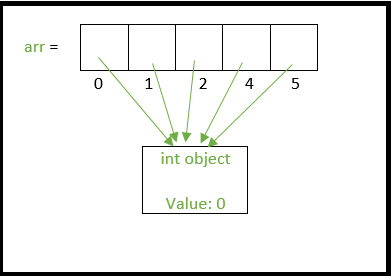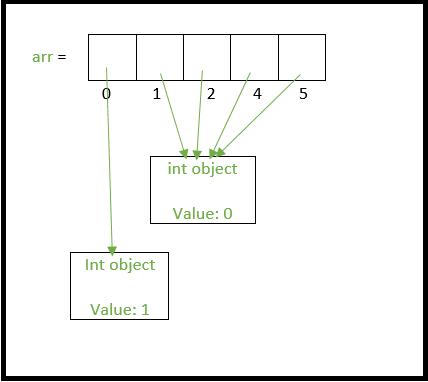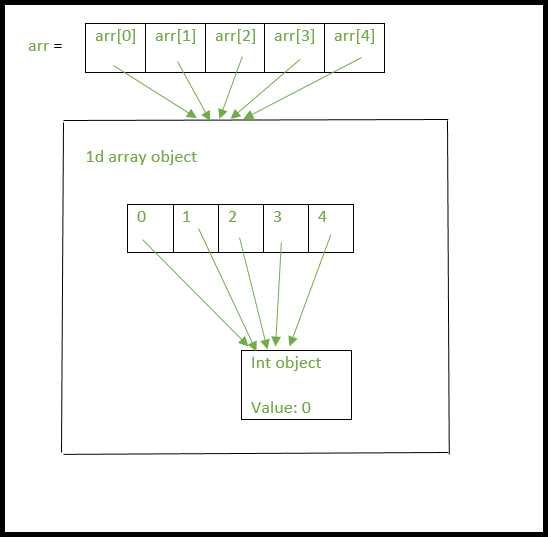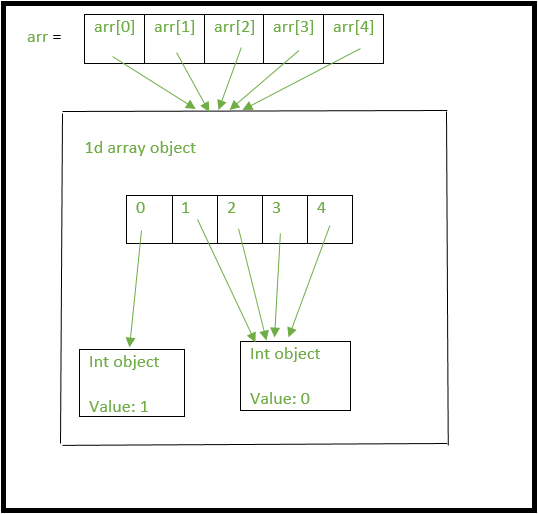GeeksforGeeks App
Open AppBrowser
Continue

# Python | Using 2D arrays/lists the right way

Python provides many ways to create 2-dimensional lists/arrays. However one must know the differences between these ways because they can create complications in code that can be very difficult to trace out. Let’s start by looking at common ways of creating a 1d array of size N initialized with 0s.

## Using 2D arrays/lists the right way

### Method 1: Creating a 1-D list

Example 1: Creating 1d list Using Naive methods

## Python3

 `N ``=` `5``ar ``=` `[``0``]``*``N``print``(ar)`

Output

`[0, 0, 0, 0, 0]`

Example 2: Creating a 1d list using  List Comprehension

## Python3

 `N ``=` `5``arr ``=` `[``0` `for` `i ``in` `range``(N)]``print``(arr)`

Output

`[0, 0, 0, 0, 0]`

Explanation:

Here we are multiplying the number of rows by the empty list and hence the entire list is created with every element zero.

### Method 2 Creating a 2-D list

Example 1: Naive Method

## Python3

 `rows, cols ``=` `(``5``, ``5``)``arr ``=` `[[``0``]``*``cols]``*``rows``print``(arr)`

Output

`[[0, 0, 0, 0, 0], [0, 0, 0, 0, 0], [0, 0, 0, 0, 0], [0, 0, 0, 0, 0], [0, 0, 0, 0, 0]]`

Explanation:

Here we are multiplying the number of columns and hence we are getting the 1-D list of size equal to the number of columns and then multiplying it with the number of rows which results in the creation of a 2-D list.

Something to be careful:

Using this method can sometimes cause unexpected behaviors. In this method, each row will be referencing the same column. This means, even if we update only one element of the array, it will update same column in our array.

## Python

 `rows, cols ``=` `(``5``, ``5``)``arr ``=` `[[``0``]``*``cols]``*``rows``print``(arr, ``"before"``)` `arr[``0``][``0``] ``=` `1` `# update only one element``print``(arr, ``"after"``)`

Output

```([[0, 0, 0, 0, 0], [0, 0, 0, 0, 0], [0, 0, 0, 0, 0], [0, 0, 0, 0, 0], [0, 0, 0, 0, 0]], 'before')
([[1, 0, 0, 0, 0], [1, 0, 0, 0, 0], [1, 0, 0, 0, 0], [1, 0, 0, 0, 0], [1, 0, 0, 0, 0]], 'after')```

Example 2: Using List Comprehension

## Python3

 `rows, cols ``=` `(``5``, ``5``)``arr ``=` `[[``0` `for` `i ``in` `range``(cols)] ``for` `j ``in` `range``(rows)]``print``(arr)`

Output

`[[0, 0, 0, 0, 0], [0, 0, 0, 0, 0], [0, 0, 0, 0, 0], [0, 0, 0, 0, 0], [0, 0, 0, 0, 0]]`

Explanation:

Here we are basically using the concept of list comprehension and applying loop for a list inside a list and hence creating a 2-D list.

Example 3: Using empty list

## Python3

 `arr``=``[]``rows, cols``=``5``,``5``for` `i ``in` `range``(rows):``    ``col ``=` `[]``    ``for` `j ``in` `range``(cols):``        ``col.append(``0``)``    ``arr.append(col)``print``(arr)`

Output

`[[0, 0, 0, 0, 0], [0, 0, 0, 0, 0], [0, 0, 0, 0, 0], [0, 0, 0, 0, 0], [0, 0, 0, 0, 0]]`

Explanation:

Here we are appending zeros as elements for number of columns times and then appending this 1-D list into the empty row list and hence creating the 2-D list.

## Python3

 `# Python 3 program to demonstrate working``# of method 1 and method 2.``rows, cols ``=` `(``5``, ``5``)``# method 2 1st approach``arr ``=` `[[``0``]``*``cols]``*``rows``# lets change the first element of the``# first row to 1 and print the array``arr[``0``][``0``] ``=` `1` `for` `row ``in` `arr:``    ``print``(row)``# outputs the following``#[1, 0, 0, 0, 0]``#[1, 0, 0, 0, 0]``#[1, 0, 0, 0, 0]``#[1, 0, 0, 0, 0]``#[1, 0, 0, 0, 0]` `# method 2 2nd approach``arr ``=` `[[``0` `for` `i ``in` `range``(cols)] ``for` `j ``in` `range``(rows)]` `# again in this new array lets change``# the first element of the first row``# to 1 and print the array``arr[``0``][``0``] ``=` `1``for` `row ``in` `arr:``    ``print``(row)` `# outputs the following as expected``#[1, 0, 0, 0, 0]``#[0, 0, 0, 0, 0]``#[0, 0, 0, 0, 0]``#[0, 0, 0, 0, 0]``#[0, 0, 0, 0, 0]`

Output

```[1, 0, 0, 0, 0]
[1, 0, 0, 0, 0]
[1, 0, 0, 0, 0]
[1, 0, 0, 0, 0]
[1, 0, 0, 0, 0]
[1, 0, 0, 0, 0]
[0, 0, 0, 0, 0]
[0, 0, 0, 0, 0]
[0, 0, 0, 0, 0]
[0, 0, 0, 0, 0]```

### Explanation:

We expect only the first element of first row to change to 1 but the first element of every row gets changed to 1 in method 2a. This peculiar functioning is because Python uses shallow lists which we will try to understand.
In method 1a, Python doesn’t create 5 integer objects but creates only one integer object and all the indices of the array arr point to the same int object as shown.If we assign the 0th index to another integer say 1, then a new integer object is created with the value of 1 and then the 0th index now points to this new int object as shown belowSimilarly, when we create a 2d array as “arr = [*cols]*rows” we are essentially extending the above analogy.

1. Only one integer object is created.
2. A single 1d list is created and all its indices point to the same int object in point 1.
3. Now, arr, arr, arr …. arr[n-1] all point to the same list object above in point 2.

The above setup can be visualized in the image below.Now lets change the first element in first row of “arr” as arr = 1

• arr points to the single list object we created we above.(Remember arr, arr …arr[n-1] all point to the same list object too).
• The assignment of arr will create a new int object with the value 1 and arr will now point to this new int object.(and so will arr, arr … arr[n-1])

This can be clearly seen in the below image.So when 2d arrays are created like this, changing values at a certain row will effect all the rows since there is essentially only one integer object and only one list object being referenced by the all the rows of the array.

As you would expect, tracing out errors caused by such usage of shallow lists is difficult. Hence the better way to declare a 2d array is

## Python3

 `rows, cols ``=` `(``5``, ``5``)``print``([[``0` `for` `i ``in` `range``(cols)] ``for` `j ``in` `range``(rows)])`

Output

`[[0, 0, 0, 0, 0], [0, 0, 0, 0, 0], [0, 0, 0, 0, 0], [0, 0, 0, 0, 0], [0, 0, 0, 0, 0]]`

This method creates 5 separate list objects, unlike method 2a. One way to check this is by using the ‘is’ operator which checks if the two operands refer to the same object.

## Python3

 `rows, cols ``=` `(``5``, ``5``)``# method 2 2nd approach``arr ``=` `[[``0` `for` `i ``in` `range``(cols)] ``for` `j ``in` `range``(rows)]``# check if arr and arr refer to``# the same object``print``(arr[``0``] ``is` `arr[``1``]) ``# prints False``# method 2 1st approach``arr ``=` `[[``0``]``*``cols]``*``rows``# check if arr and arr refer to``# the same object``# prints True because there is only one``# list object being created.``print``(arr[``0``] ``is` `arr[``1``])`

Output

```False
True```

My Personal Notes arrow_drop_up